A random sample of 900 13- to 17-year-olds found that 411 had a computer in their room with Internet access. Let p be the proportion of all

Question

A random sample of 900 13- to 17-year-olds found that 411 had a computer in their room with Internet access. Let p be the proportion of all teens in this age range who have a computer in their room with Internet access.Suppose you wished to see if the majority of teens in this age range have a computer in their room with Internet access. To do this, you test the hypotheses
H0: p = 0.50 vs HA : p≠0.50
The test statistic for this test is:_______.
a. 1.96
b. 2.60
c. 27.50
d. 2.59424

in progress 0
5 months 2021-08-27T15:28:54+00:00 1 Answers 0 views 0

The test statistic for this test is: z = -2.60, option b.

Step-by-step explanation:

The test statistic is: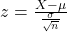In which X is the sample mean,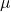is the value tested at the null hypothesis,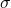is the standard deviation and n is the size of the sample.

H0: p = 0.50 vs HA : p≠0.50

This means that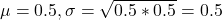A random sample of 900 13- to 17-year-olds found that 411 had a computer in their room with Internet access.

This means that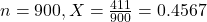Value of the test-statistic: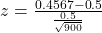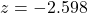The test statistic for this test is: z = -2.60, option b(should be).